# Understanding linear regression

4.3Towards the end of the previous chapter, we concluded with a mention of linear regression. Remember? Let’s pick things up from there and understand how linear regression can help you make sense of the relationship between two variables in a huge dataset. First, we’ll take a look at the meaning of regression.

### Understanding the ‘regression’ in linear regression

To understand linear regression, let’s begin by making sense of the two words involved - linear and regression. Linear, as you know, means resembling a line. Regression simply means the act of going back to a previous place or state of existence.

In fact, the term ‘regression’ was coined by Francis Galton in the 19th century. He used it to describe a very fascinating biological phenomenon. Galton noted that  tall parents generally tend to have tall children, but those tall children are nevertheless shorter than their parents. Meanwhile, short parents mostly tend to have short children, but those short children are taller than their parents. He called this ‘regression towards mediocrity.’ It’s a reversion to the average human height.

Here’s a pictorial representation of this phenomenon that Galton studied.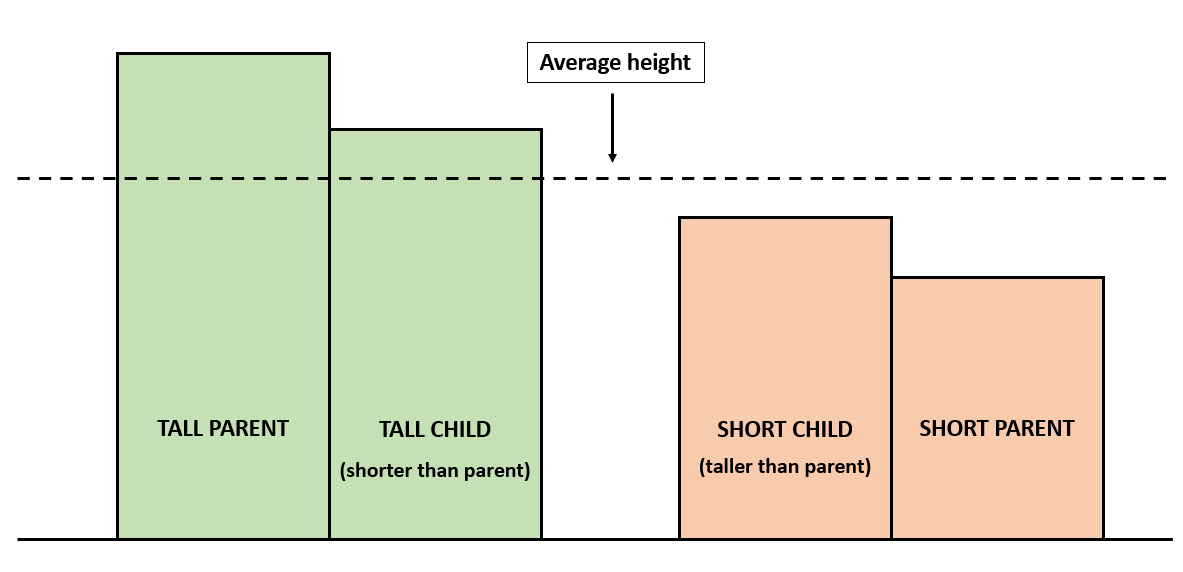### What is linear regression, then?

So, what does linear regression mean? Combining the meanings, it must point to the act of going back to a state of linearity - a state of line-like pattern. Now, what does this have to do with all the data we’ve been dealing with? Let’s delve into that.

Do you recall the example we saw in the previous chapter, of the samosas and their prices? In that example, there was a clear, logical relationship between the number of samosas bought (x) and the money spent (y). So, finding out the slope of the equation and the intercept, and establishing the relationship between those two variables was a piece of cake.

But, when you take a year’s worth of data related to the price of two stocks, as you may likely do when you’re trying to set up a pair trade, there’s no clear pattern that you can observe. The prices of the two stocks are scattered all over the place, and there is no predetermined relationship between the two stocks. Neither is dependent on the other, technically.

So, if you wish to apply the straight line equation to the prices of the pair of stocks you’re working with, you’re going to need the help of a statistical tool created precisely for this purpose - linear regression.

Linear regression is a technique that attempts to figure out the relationship between two variables by fitting a linear equation to the given data. Note the words ‘fitting a linear equation.’ They mean that since the data is discrete and unconnected, the linear regression technique tries to make sense of the data points you have and identify a possible relationship between them.

### Linear regression: Plotting the data points

Take the prices of two stocks - TCS and Infosys. As we saw in the previous chapter, here’s a small snippet of their prices over a 10-day period.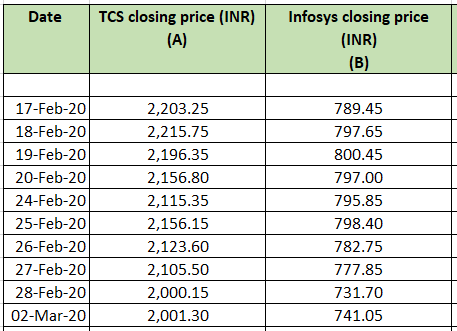Clearly, there’s no decipherable relationship between these numbers. In fact, if you plot this data on a graph, the price points will be all over the place. This is even more true in case of large volumes of data.

To give you a better idea of how unrelated data may appear on a graphical plane, here’s a picture.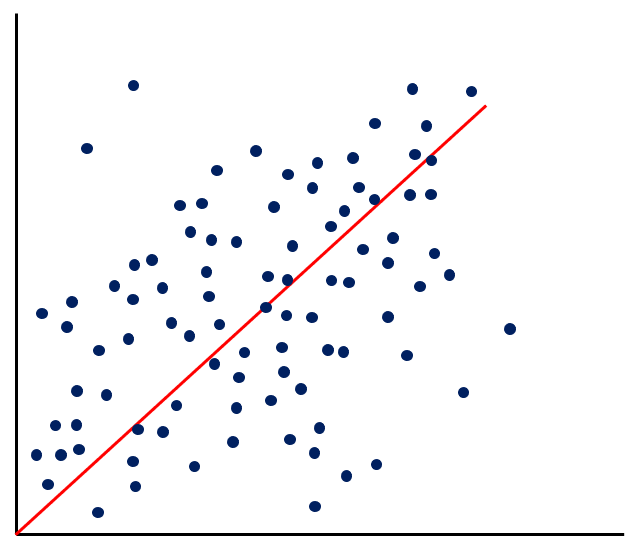The red line that you see - that’s the result of linear regression. It attempts to find the straight line equation that is true for a majority of the data points in the given set.

That straight line is the red line shown in the image above.

### Calculating linear regression

Is there a formula to calculate linear regression? Sure, and you may even have learned it in school. But when you’re dealing with huge volumes of data, it’s tedious to make manual calculations. Fortunately, we have an MS Excel plugin that works efficiently enough. With just a few clicks, you can be on your way to the thick of regression analysis.

### Running the regression function

Under the data tab in your excel sheet, click the data analysis option and choose ‘regression.’ You will see a window that asks you to input the details of the x and y variables. In this case, they will be the prices of each stock. For the sake of continuity, let’s take up TCS and Infosys shares over the same 1-year period we’ve been dealing with.

Here’s what the input for the linear regression function looks like.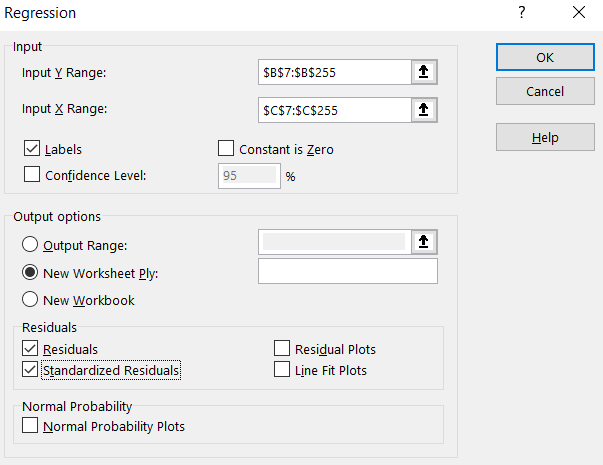A few things to note here:

• Input Y range here is the column with TCS share prices.
• Input X range here is the column with Infosys share prices.

Does this mean TCS share prices depend on Infosys share prices? No. It doesn’t mean that. We’ve simply taken it this way because of the order in which the data has been arranged. It can also be taken the other way around, with the Infosys share prices forming the input Y range and the TCS share prices forming the input X range.

But which share should we consider as the dependent one, and which as the independent one? We’ll get to that in a bit. For now, let’s get on with the regression analysis.

Once you’ve checked the ‘residuals’ and the ‘standardized residuals’ boxes, click OK.

### Linear regression function: The output

This is what the output will look like.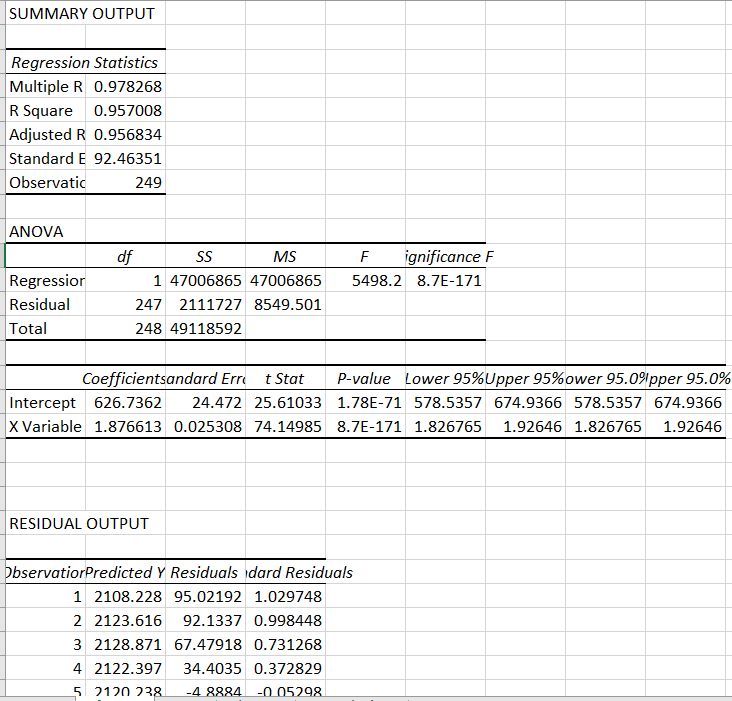There’s a lot of information here, undoubtedly. But for now, let’s concern ourselves with the two values we need to form the straight line equation (which is kind of the main point of running this function).

We need to identify the slope and the intercept. Fortunately, you don’t have to do much searching. The output shows you the intercept and the slope directly. Check it out here.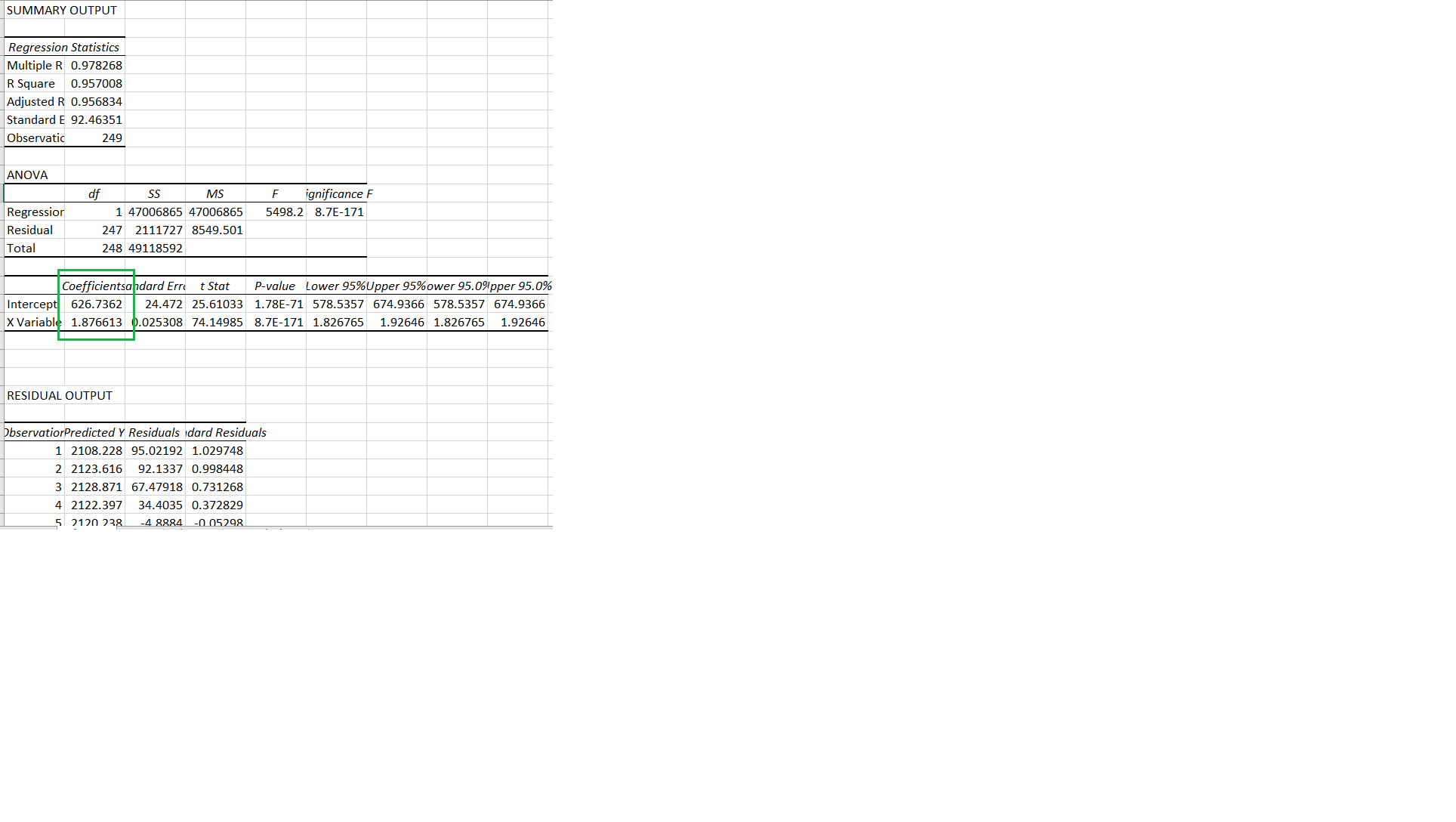The intercept for the data set we took is 626.74.

The slope is 1.8766.

So, the equation we’re looking at is: y = 1.8766x + 626.74

### Residuals

Figuring out the equation is only one thing. Does it mean that every pair of TCS and Infosys share prices will fit into that equation? Certainly not. In fact, it’s highly unlikely that any pair of the prices will fit into the equation perfectly.  The reason for this lies in the essence of linear regression.

Earlier in this chapter, we saw that linear regression is a technique that attempts to figure out the relationship between two variables by fitting a linear equation to the given data. Again, note the words ‘fitting a linear equation.’ They essentially mean that the technique takes existing data and attempts to fit it all into a common straight line equation.

Let’s take a relatable example to understand this better. Say you’re playing around with some clay. You have a star-shaped mold. And you want to use some clay to create a star-shaped clay model. So, you take a handful of clay and attempt to fit it into the mold. Now, since the clay has no shape by itself, and since you’re trying to fit it into a predetermined pattern, some of it may spill over outside the mold. You remove this excess clay and keep only what you need to make the star.

In linear regression, this excess is what the residuals are all about. It represents the part of a variable that does not fit into the straight line equation. To put it differently, it shows you how far a particular data point is from the regression line.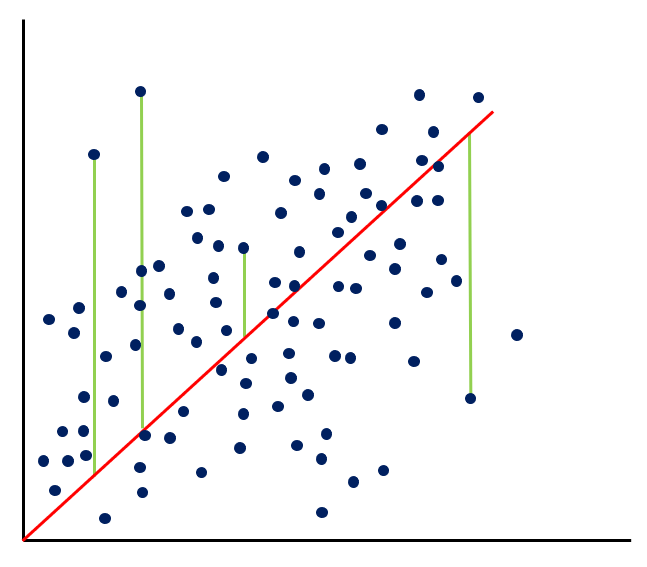See the green vertical lines connecting some of the data dots to the regression line? That is how the residuals are represented on the graph. In the linear regression function output, you’ll find them here.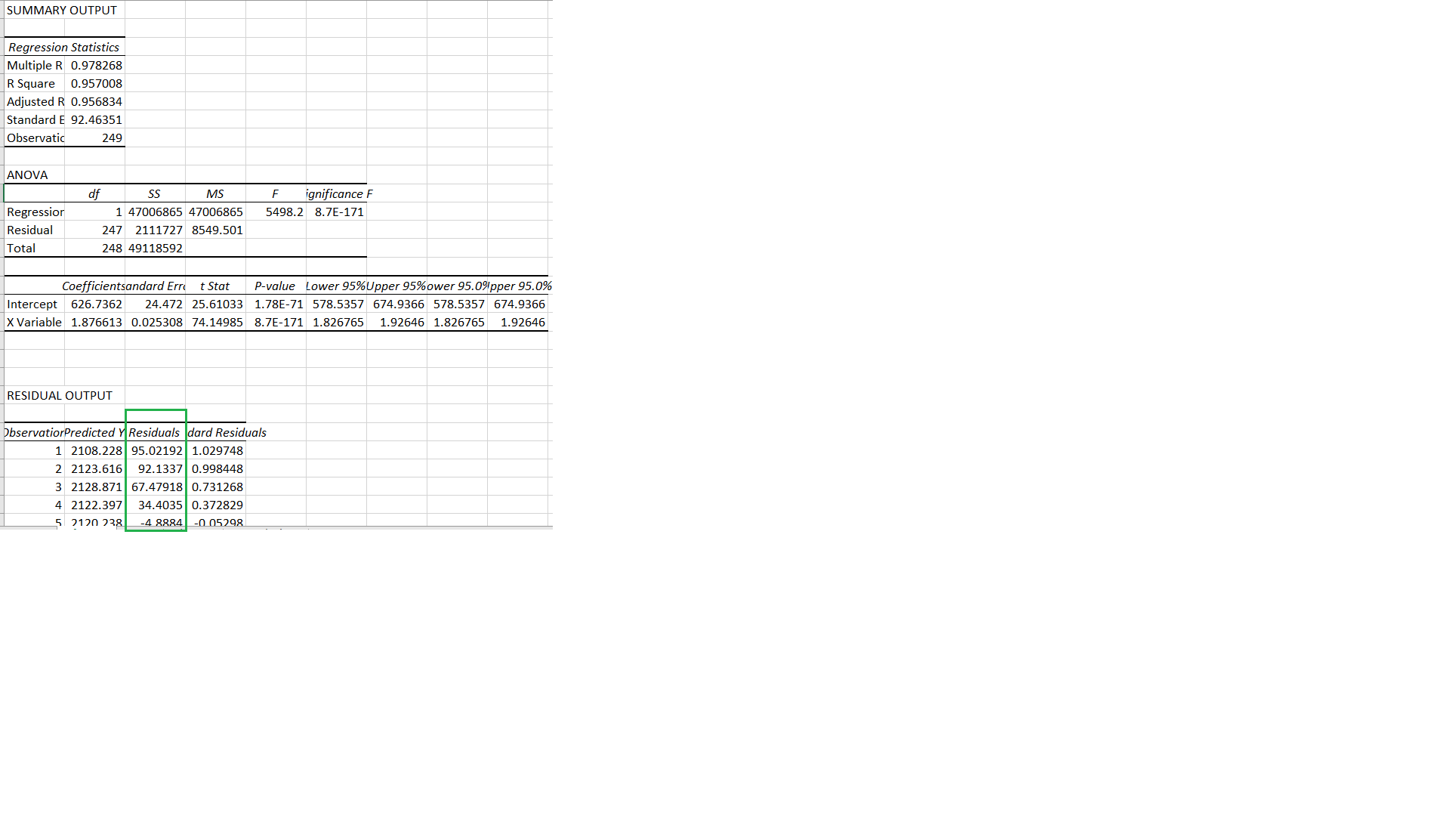Since we’ve taken the 1-year data for TCS and Infosys shares, we have around 249 pairs of stock prices. Each pair will likely have a residual, unless it falls exactly on the regression line, in which case the residual will be zero.

#### Wrapping up

So, why do we calculate the residuals? Well, as you saw in one of the images above, the residuals give you the distance between the variables and the regression line. The higher the residual, the further away the variable is from the average. So, the higher the chances of it reverting to the mean, just like we saw with the first method of pair trading. In other words, when the residual is between -3 SD and -2 SD or between 2 SD and 3 SD, that may be a trigger for a pair trade.

#### A quick recap

• Linear regression points to the act of going back to a state of linearity - a state of line-like pattern.
• It is a technique that attempts to figure out the relationship between two variables by fitting a linear equation to the given data.
• We have an MS Excel plugin that works efficiently enough. With just a few clicks, you perform regression analysis.
• The linear regression output shows you the intercept and the slope directly.
• It also shows you the residuals, which show you how far a particular data point is from the regression line.
• The higher the residual, the further away the variable is from the average. So, the higher the chances of it reverting to the mean.
• When the residual is between -3 SD and -2 SD or between 2 SD and 3 SD, that may be a trigger for a pair trade.